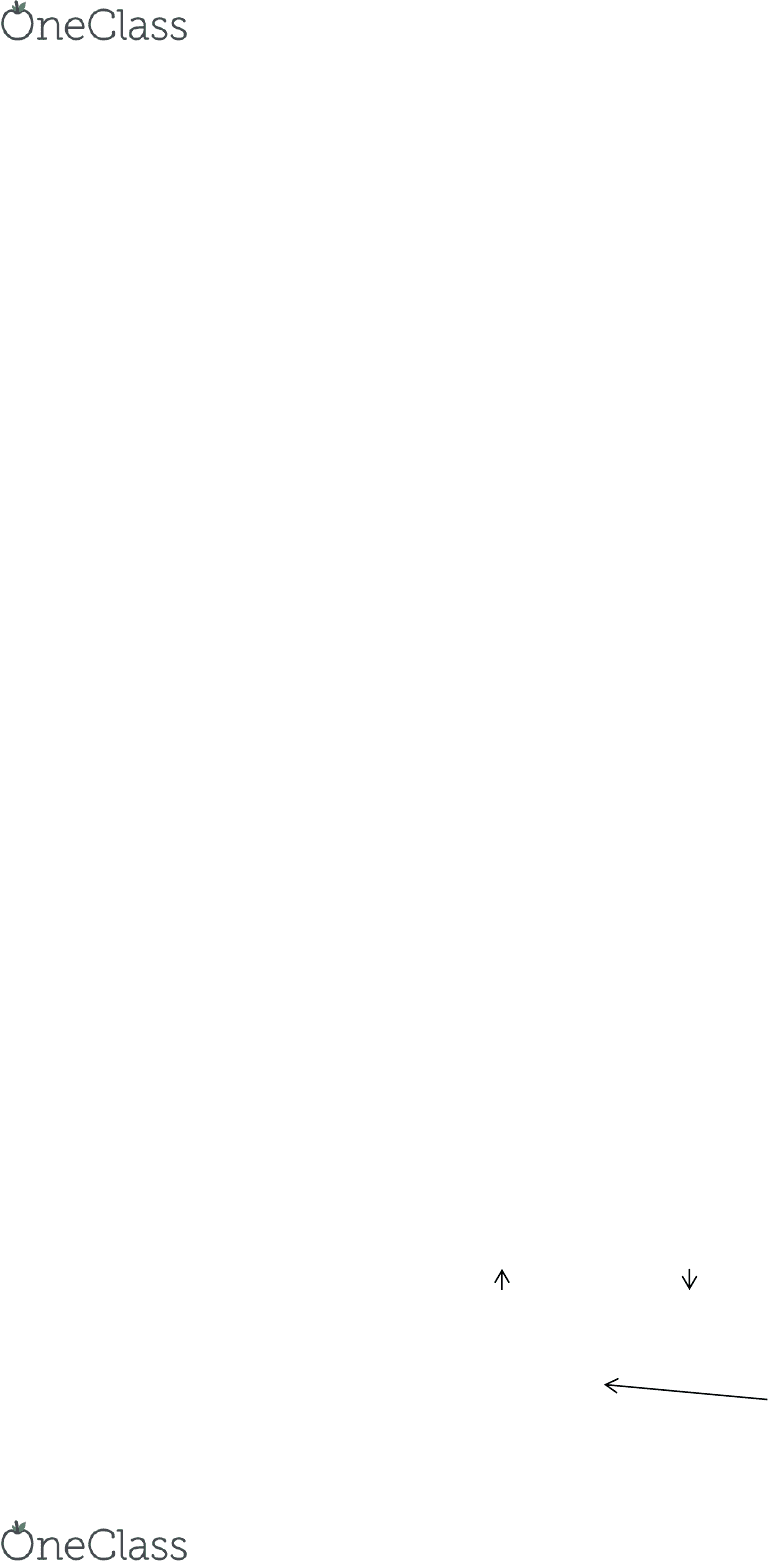Textbook Notes (280,000)
CA (170,000)
McMaster (10,000)
COMMERCE (2,000)
Chapter 7

# COMMERCE 1AA3 Chapter Notes - Chapter 7: Book Value, Ddb Worldwide

Department
Commerce
Course Code
COMMERCE 1AA3
Professor
Chapter
7

This preview shows half of the first page. to view the full 3 pages of the document.FINAL EXAM NOTES: Chapter 7
Tangible Assets:
Physical substance property, plant & equipment, fixed assets
Intangible Assets:
Assets:
Tangible asset measured at acquisition costs
Some intangible subject to amortization
Land:
Purchase price
Commissions
Survey & legal fees
Back property taxes paid
Several assets purchased in a group at one price
Total cost allocated based on market value
Assets market value ÷ total market value = % of total market value x total cost
= cost of each asset
Subsequent Costs:
Two types of expenditures
o Capital Expenditure increase productive life, operating efficiency, capacity of asset
Cost added to an asset account
o Revenue Expenditure maintain productive capacity of the asset
Cost recorded as an expense
Depreciation Methods:
1. Straight-Line annual depreciation = depreciable cost ÷ useful life
o Accumulated depreciation = carrying amount (residual value)
2. Double Diminishing beginning book value x depreciation rate (2 ÷ useful life)
o First year depreiation ased on asset’s full ost
3. Sum-of-the-years-digits depreciable cost x (n ÷ SYD)
o n = useful life in years / SYD (consistent) = (n x (n+1)) ÷ 2 (n decreases by one per year)
find more resources at oneclass.com
find more resources at oneclass.com